# LOG#164. Theta functions.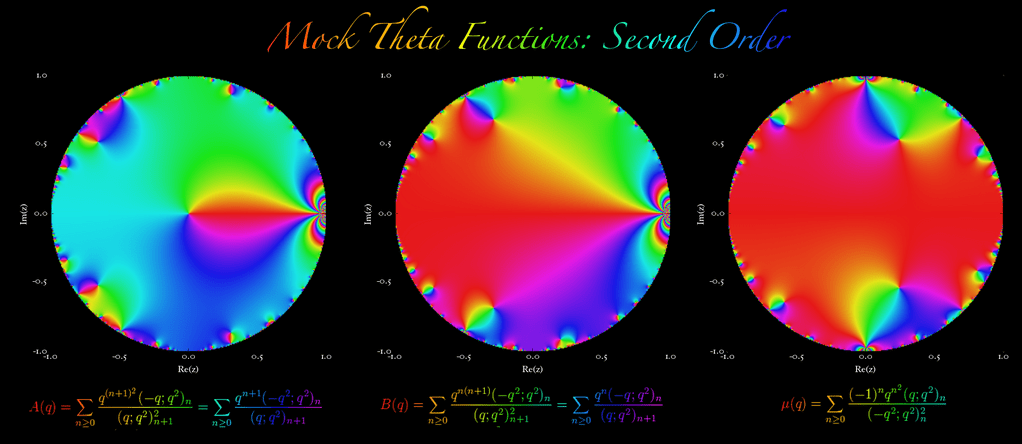Hi, there! We are going to explore more mathematical objects in this post. Today, the objects to study are theta functions. A prototype is the Jacobi theta function:

(1)whereand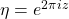. It satisfies the functional equation

(2)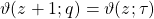and

(3). The Jacobi theta function is related to the Riemann zeta function. With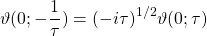we have

(4)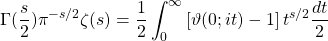We can also define some auxiliary Jacobi theta functions that we found in the classical literature about this complex subject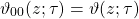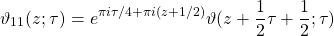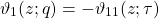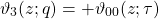whereis sometimes called the nome. Auxiliary theta functions can be written in terms of the nome, and ifthey satisfy the following Jacobi identities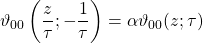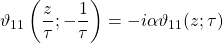The Jacobi theta function is the fundamental solution to the 1D heat equation with spatially periodic boundary conditions. That is, if we write:we find that it verifies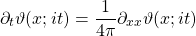withSo, the Jacobi theta function becomes the Dirac comb when t approaches to zero. Some very interesting values of the Jacobi theta function are related to the gamma function: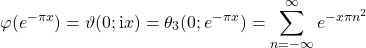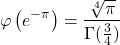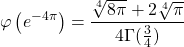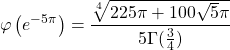The notion of theta function can be generalized. Let F be a quadratic form in n-variables. Then, we defineIt is a modular form of weight n/2. The Ramanujan theta function arises in a similar way as well:

(5)and whereis a n-dimensional complex vector, T denotes the transpose,, such as. Note that the n-dimensional analogue of the modular group is the sympletic group. And the double cover of the symplectic group is the metaplectic group. It can be defined over either real or p-adic numbers. The construction covers more generally the case of an arbitrary local or finite field, and even the ring of adeles.

The metaplectic group has a particularly significant infinite-dimensional linear representation, the Weil representation. It was used by André Weil to give a representation-theoretic interpretation of theta functions, and is important in the theory of modular forms of half-integral weight and the theta correspondence.

The fundamental group of the symplectic Lie groupis infinite cyclic, so it has a unique connected double cover, which is denoted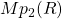and it denotes the metaplectic group.

The metaplectic groupis not a matrix group: it has no faithful finite-dimensional representations. Therefore, the question of its explicit realization is nontrivial. For more details, and the completion of all this material, you can read the whole article about the metaplectic group in the wikipedia.

The Ramanujan theta function satisfies a beautiful functional equation

(6)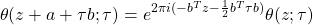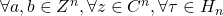A much more sophisticated (and modern) concept of theta function is that of mock theta function and mock modular forms.But it will be the topic of a future post…Some day.

See you in my next blog post!!!!!

This site uses Akismet to reduce spam. Learn how your comment data is processed.